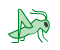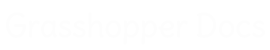﻿ Tween Through Curves On Surface - Pufferfish - Component for Grasshopper | Grasshopper DocsCommunity documentation for Grasshopper add-ons & pluginsColor
 Curve
 Domain
 List
 Mesh
 Number
 Plane
 Point
 SubD
 Surface
 Transform
 Twisted Box
 Vector

# Tween Through Curves On Surface

## Inputs

NameIDDescriptionType
CurvesCSet of curves on surface to tween throughCurve
SurfaceSSurface to tween curve onSurface
FactorFTween factor (0.0 = Curve at C start, 1.0 = Curve at C end), unless Normalized is FalseNumber
NormalizedNIf False, the rules for factor are (0.0 = Curve at index 0, Index number of last curve = Curve at last index) The tween factor range from one curve to the next curve in the list is: Index number of a curve = the curve at that index, Index number of the next curve = the next curve in the list, factors between the curve index numbers will be the corresponding tweens. For instance: A tween factor of 2.5 will result in a tween curve halfway between the curve at index 2 and the curve at index 3.Boolean
EqualizedEIf True, tween distribution is evaluated along the tween path without considering the locations of the input curve setBoolean
LoopbackLIf True, allow the tween path to continue from the end back to the start as a closed loop (if Normalized is False, increase the tween factor range maximum by 1)Boolean
Interpolation TypeIDetermines how the tween path is interpolated 0 = Straight 1 = Normal 2 = Tight 3 = UniformInteger
RefitROptional Refit match method. (No Integer or 0 = Off, Integer greater than 0 = On and curve degree of refit) If an integer greater than zero, Refit match method is used if possible. When input curves are refit their control points are redistributed, added to, and removed from based on the curves curvature and the input integer degree, while trying to maintain their shapes. Refit results in tighter shaped tweens, with curvature based control point distribution.Integer
Point SamplePOptional Point Sample match method. (No Integer or 0 = Off, Integer greater than 0 = On and amount of sample points) If an integer greater than zero, Point Sample match method is used. When input curves are sampled their control points are recreated by equally dividing the curve by the input integer point count. Point Sample results in looser shaped tweens, with uniform control point distribution.Integer

## Outputs

NameIDDescriptionType
TweenTResulting tween curve on surfaceCurve

## Video Tutorials

Site design © Robin Rodricks.   Pufferfish and associated data © 2023 Michael Pryor.
Rhinoceros and Grasshopper are registered trademarks of Robert McNeel & Associates.  Hosted by GitHub